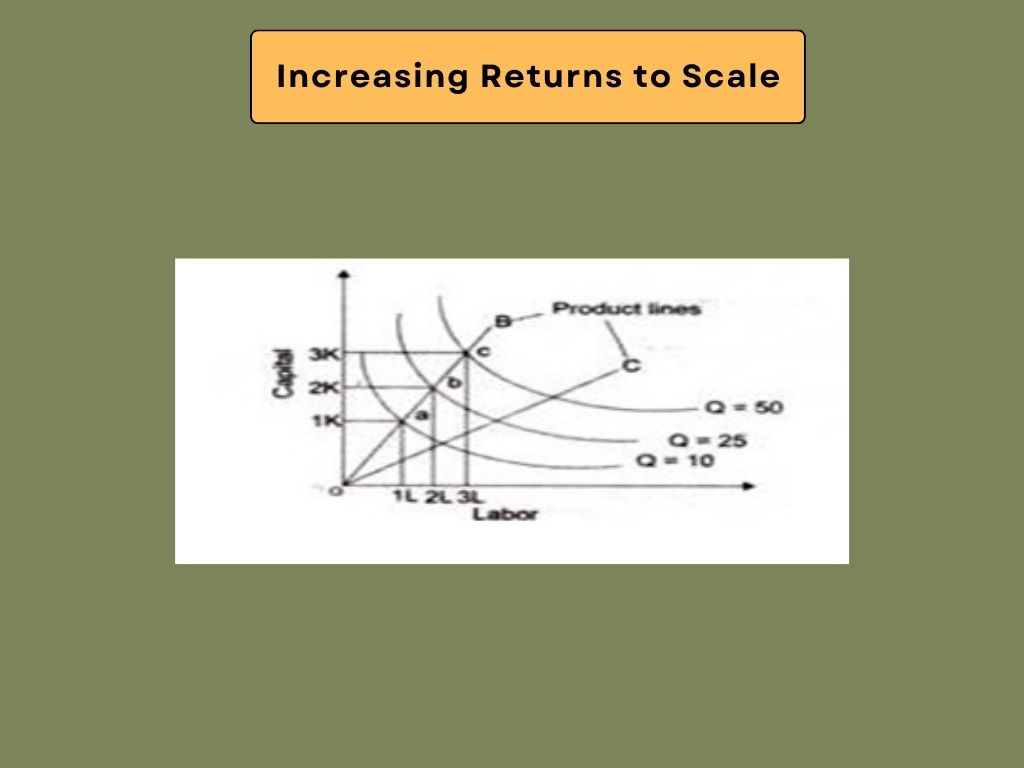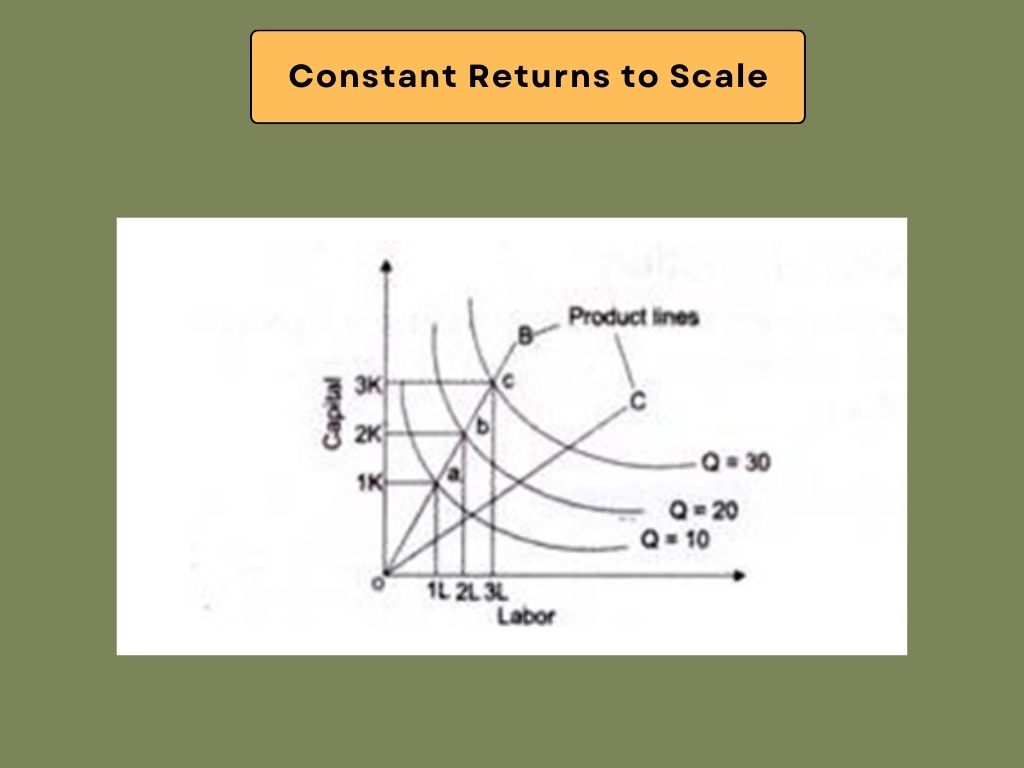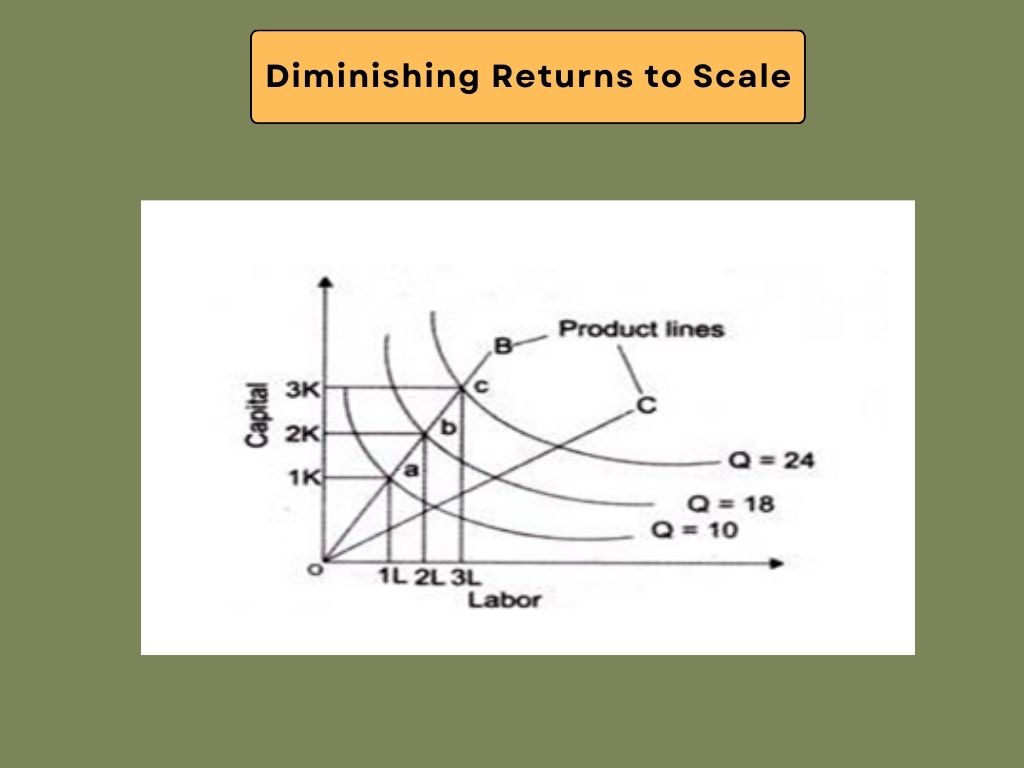Management Notes

# Management Notes

Reference Notes for Management

# Law of Returns to Scale – Introduction to Microeconomics | Management Notes

## Definition of Law of Returns to Scale

According to the law of returns to scale, output changes in proportion to input changes. The law of returns to scale states that when there is a proportionate change in input, the output also changes. Every factor of production is variable over the long term. There is no fixed factor. Thus, changing the quantity of all factors of production can change the scale of production. The distinction between fixed factors and variable factors vanishes in the long run. This means that in the long-run everything is variable.

As inputs are increased in the same proportion, the law of returns to scale describes the relationship between output and input scale in the long run. As a result of the law of returns to scale, when the amount of inputs changes proportionately, the output also changes. Changes in inputs influence output in different ways. As an example, output changes by a large, same, or small proportion based on changes in input.

## Assumptions of Law of Returns to ScaleSome of the assumptions of law of returns to scale are as follows:

i. The factors of production (land, labor, capital and organization) are all variable.
ii. Law assumes constant technological advancement. This means that there is no technological change over the course of time considered.
iii. There is a perfect level of competition on the market.
iv. The outputs or returns are measured in physical terms.
v. It is based on long-term analysis.

## Types of Law of Returns to Scale

When factor inputs are used proportionately, i.e. increasing the production scale, output may increase more than proportionately, increase at the same rate as inputs, or increase less than proportionately (not assumed to decrease). The law of returns to scale explains this. As a result, there are three possible cases of scale returns: increasing scale returns, constant scale returns, and decreasing scale returns.

1)  Increasing Returns to Scale
2) Constant Returns to Scale
3)  Diminishing Returns to Scale### 1)  Increasing Returns to Scale:

The proportional change in the output of an organization is greater than the proportional change in inputs, and the production is said to reflect increasing returns to scale. In order to produce a particular product, doubling the quantity of inputs and increasing the output by more than twice is said to be an increasing returns to scale. As the scale of production increases, the cost per unit produced decreases. When economies of scale are in place, the average cost per unit produced is lower.Figure: Increasing Returns to Scale

The above figure shows that a movement from a to b represents a doubling of input. Now, the combination of inputs reaches 2K+2L from 1K+1L. In spite of this, the output increased from 10 to 25 (150% increase), which is more than twice as much. In the same way, when input changes from 2K-H2L to 3K + 3L, output changes from 25 to 50 (a 100% increase), which is greater than the change in input. This clearly shows the increasing returns to scale.

#### Factors Affecting Increasing Returns to Scale

Increased returns to scale can be attributed to many factors. Some of the factors that are responsible for increasing returns to scale are as follows:

a) Indivisibility of managerial and technical functions: The inputs, such as machines and human resources, used for the production process are fixed. It is not possible to divide these inputs according to production levels. As an example, an organization cannot use half a turbine for small-scale production. In the same vein, an organization cannot use half of a manager to achieve small scale production. As a result of this technical and managerial indivisibility, an organization needs to employ the minimum quantity of machines and managers, even if production levels are far below their capacity. Therefore, if inputs are increased, output is also increased exponentially.

b)  Specialization: A high degree of specialization of man and machine facilitates the scaling up of production. It is possible to increase the productivity of labor and capital per unit through the use of specialized labor and machinery. As a result, it shows increasing returns to scale.

c)  Concept of Dimensions: The concept of dimensions is related to the concept of increasing returns to scale. Increasing the length and breadth of a room will more than double its area, according to the principle of dimensions. An example of this is the increase in length from 15 to 30 and in breadth from 10 to 20. Thus, length and width are doubled. If so, the area of the room would increase from 150 (15*10) to 600 (30*20), more than doubling.

a. Specialization and Division of Labor
b. External Economies expanded by the industry
c. Effects of internal as well as external economies
d. With an increase in individual factors of production, such as machines and management techniques, an increase in output is produced.

### 2)  Constant Returns to Scale:

It is said that a production generates constant returns to scale when a proportionate change in input corresponds to a proportionate change in output. An example of constant returns to scale is when inputs are doubled, so output should also be doubled.Figure: Constant Returns to Scale

The above figure shows that input doubles whenever there is a movement from a to b. The output now increases from 10 to 20 when the combination of inputs reaches 2K+2L from IK+IL. In the same way, if the input changes from 2Kt2L to 3K + 3L, then the output changes from 20 to 30, which is equal to the input change. This demonstrates constant returns to scale. Constant returns to scale have divisible inputs and homogeneous production functions.

a. A level of output cannot be reached when economies and diseconomies of scale do not work together.
b. The combination of indivisible factors is optimal when other factors are indivisible.

A diminishing return to scale occurs when a proportionate increase in output is less than a proportionate increase in input. As an example, when capital and labor are doubled but the output is less than doubled, the returns to scale would be described as diminishing.The above figure shows that when labor and capital are combined from point a to point b, input is doubled. An input combination of 1k+1L occurs at point a, and a combination of 2K+2L occurs at point b. Although the output has increased from 10 to 18, the change in the amount of input is less. If input is changed from 2K+2L to 3K+3L, then output changes from 18 to 24, which is less than change in input. This illustrates diminishing returns to scale.

The diminishing returns to scale are due to the diseconomies of scale that arise from managerial inefficiency. Large organizations, in general, suffer from managerial inefficiency. Resources are also at a premium in large-scale organizations. The number of coal mining plants in a company can be increased, but output cannot be increased due to limited coal reserves.

a. When prices of raw material rises.
b. When there is increment of price of factors.
c. When a factor becomes inefficient and ineffective.
d. The difficulties in supervision and coordination of factors make managing the firm difficult.
e. Excessive competition among firms for factors of production and raw materials.

Essentially, both economics and diseconomies of scale are simultaneously operating in production regardless of the size of the production. Whenever economies of scale outweigh diseconomies of scale, IRS emerges. When economies of scale are outweighed by diseconomies of scale, DRS results. In the absence of diseconomies of scale, CRS emerges. According to empirical studies about the law of returns to scale, IRS operates at an initial stage or small-scale, CRS operates at a medium scale, and DRS operates at a large scale. In this comparison, CRS was found to have existed for a longer period of time than others.

According to some economists, entrepreneur is the fixed factor, which cannot be used in the fixed proportion with other factors. According to this view, the law of returns to scale is a special case of the law of variable proportion because the entrepreneur is a fixed factor, while the rest of others are variable. There has been a challenge in the operation of the law of returns to scale with this argument. Even if we consider entrepreneurship to be the human effort rather than the number of entrepreneurs, this law certainly appears logical and operational since entrepreneurial effort directly varies with the scale of operations regardless of the number of entrepreneurs.

## Formal Definitions Law of Returns to Scale

According to formal definitions, a production function F(K,L) is defined to have:

a. Constant returns to scale if (for any constant a greater than 0) F(aK , aL)= aF(K , L) (Function F is homogeneous of degree 1)
b. Increasing returns to scale if (for any constant a greater than 1) F(aK , aL) > aF(K ,L)
c. Decreasing returns to scale if (for any constant a greater than 1) F(aK , aL)< aF(K , L)
where K and L are factors of production—capital and labor, respectively.

In a more general setting, for a multi-input-multi-output process, one can assume technology can be represented by a technology set, T, that must satisfy certain regularity criteria associated with production theory. A constant returns to scale property is equivalent to saying that technology set T is a cone, i.e., it satisfies the property aT=T, a>0. The production function that describes the technology set T must also be homogeneous of degree 1.

## Example Related to Law of Returns to Scale

In the assumption that factor costs are constant (i.e., that the firm is equally competitive in all input markets) and that the production function is homothetic, a firm experiencing constant returns will have constant long-run average costs, a firm experiencing decreasing returns will have increasing long-run average costs, and a firm experiencing increasing returns will have decreasing long-run average costs. The relationship breaks down, however, if the firm does not face perfectly competitive factor markets (i.e., in this context, the price for a good does depend on what is purchased).

If, for example, there are increasing returns to scale in some ranges of output levels, but the firm is so large in one or more input markets that increasing its purchases of an input raises its per-unit cost, then the firm could suffer from diseconomies of scale in that range. However, if the company is able to get bulk discounts on an input, it could have economies of scale in some range of output levels even if it has decreasing returns on production in that range.